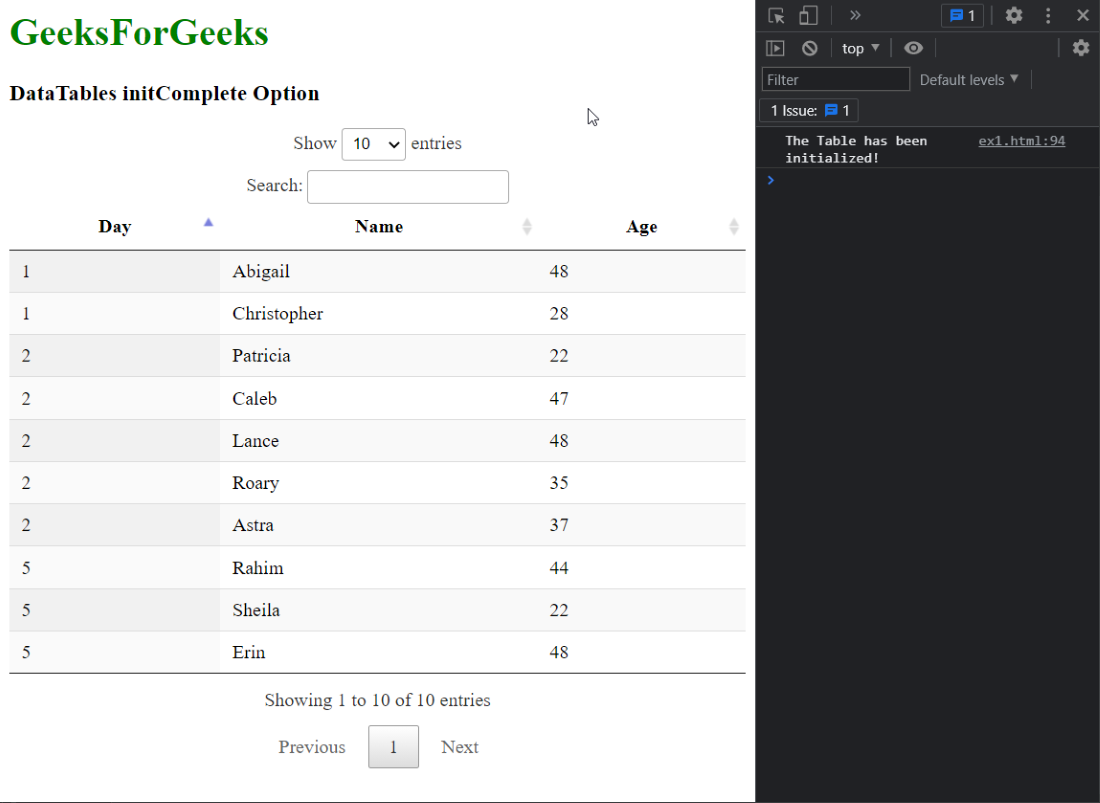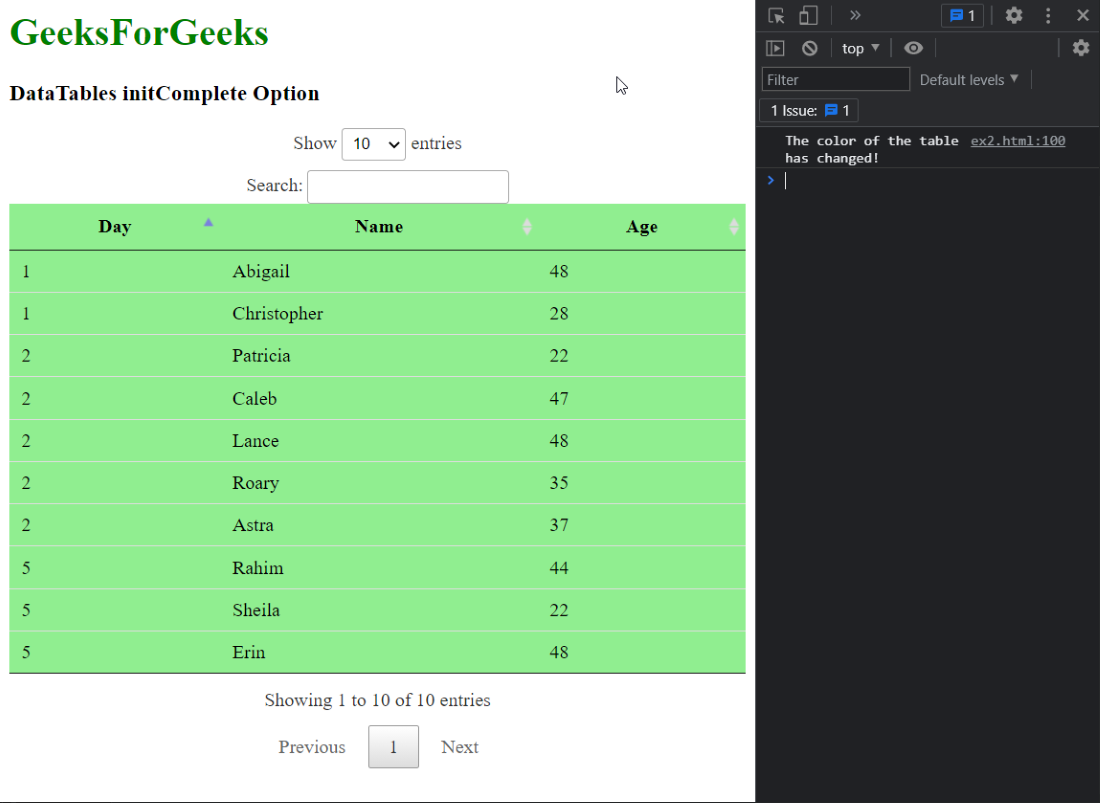Open in App
Not now

# DataTables initComplete Option

• Last Updated : 11 Sep, 2021

DataTables is jQuery plugin that can be used for adding interactive and advanced controls to HTML tables for the webpage. This also allows the data in the table to be searched, sorted, and filtered according to the needs of the user. The DataTable also exposes a powerful API that can be further used to modify how the data is displayed.

The initComplete option is used to specify the function that will be invoked when the DataTable has fully loaded all the data. This can be useful information in situations where one might require to modify the table in any manner after the data has loaded. It has the JSON parameter as a callback that returns the data that was returned by the server.

Syntax:

`function initComplete( settings, json )`

Parameters: This option has a two parameters as mentioned above and described below:

• settings: This is the settings object of the DataTable containing all the settings with which the table was initialized.
• json: This is the JSON data that is retrieved from the server when the ajax option is used. It is undefined when this option is not used.

The examples below illustrate the use of this option.

Example 1: In this example we use the initComplete callback to log to the console that the table has been initialized.

## HTML

 ```<``html``>``<``head``>``  ````  ``<``script` `type``=``"text/javascript"` `          ``src``=``"https://code.jquery.com/jquery-3.5.1.js"``>``  ```` ` `  ````  ``<``link` `rel``=``"stylesheet"``        ``href``=``"https://cdn.datatables.net/1.10.23/css/jquery.dataTables.min.css"``>`` ` `  ````  ``<``script` `src``=``"https://cdn.datatables.net/1.10.23/js/jquery.dataTables.min.js"``>``  ```` ` ```<``body``>``  ``<``h1` `style``=``"color: green;"``>``    ``GeeksForGeeks``  ````  ``<``h3``>DataTables initComplete Option`` ` `  ````  ``<``table` `id``=``"tableID"` `class``=``"display nowrap"``>``    ``<``thead``>``      ``<``tr``>``        ``<``th``>Day``        ``<``th``>Name``        ``<``th``>Age``      ````    ````    ``<``tbody``>``      ``<``tr``>``        ``<``td``>2``        ``<``td``>Patricia``        ``<``td``>22``      ````      ``<``tr``>``        ``<``td``>2``        ``<``td``>Caleb``        ``<``td``>47``      ````      ``<``tr``>``        ``<``td``>1``        ``<``td``>Abigail``        ``<``td``>48``      ````      ``<``tr``>``        ``<``td``>5``        ``<``td``>Rahim``        ``<``td``>44``      ````      ``<``tr``>``        ``<``td``>5``        ``<``td``>Sheila``        ``<``td``>22``      ````      ``<``tr``>``        ``<``td``>2``        ``<``td``>Lance``        ``<``td``>48``      ````      ``<``tr``>``        ``<``td``>5``        ``<``td``>Erin``        ``<``td``>48``      ````      ``<``tr``>``        ``<``td``>1``        ``<``td``>Christopher``        ``<``td``>28``      ````      ``<``tr``>``        ``<``td``>2``        ``<``td``>Roary``        ``<``td``>35``      ````      ``<``tr``>``        ``<``td``>2``        ``<``td``>Astra``        ``<``td``>37``      ````    ````  ````  ``<``script``>`` ` `    ``// Initialize the DataTable``    ``\$(document).ready(function () {``      ``\$('#tableID').DataTable({`` ` `        ``initComplete: function () {``          ``console.log("The Table has been initialized!")``        ``}``      ``});``    ``}); ``  ```````

Output:Example 2: In this example we use the callback to change the color of the table after it is initialized.

## HTML

 ```<``html``>``<``head``>``  ````  ``<``script` `type``=``"text/javascript"` `          ``src``=``"https://code.jquery.com/jquery-3.5.1.js"``>``  ```` ` `  ````  ``<``link` `rel``=``"stylesheet"``        ``href``=``"https://cdn.datatables.net/1.10.23/css/jquery.dataTables.min.css"``>`` ` `  ````  ``<``script` `src``=``          ``"https://cdn.datatables.net/1.10.23/js/jquery.dataTables.min.js"``>``  ```` ` ```<``body``>``  ``<``h1` `style``=``"color: green;"``>``    ``GeeksForGeeks``  ````  ``<``h3``>DataTables initComplete Option`` ` `  ````  ``<``table` `id``=``"tableID"` `class``=``"display nowrap"``>``    ``<``thead``>``      ``<``tr``>``        ``<``th``>Day``        ``<``th``>Name``        ``<``th``>Age``      ````    ````    ``<``tbody``>``      ``<``tr``>``        ``<``td``>2``        ``<``td``>Patricia``        ``<``td``>22``      ````      ``<``tr``>``        ``<``td``>2``        ``<``td``>Caleb``        ``<``td``>47``      ````      ``<``tr``>``        ``<``td``>1``        ``<``td``>Abigail``        ``<``td``>48``      ````      ``<``tr``>``        ``<``td``>5``        ``<``td``>Rahim``        ``<``td``>44``      ````      ``<``tr``>``        ``<``td``>5``        ``<``td``>Sheila``        ``<``td``>22``      ````      ``<``tr``>``        ``<``td``>2``        ``<``td``>Lance``        ``<``td``>48``      ````      ``<``tr``>``        ``<``td``>5``        ``<``td``>Erin``        ``<``td``>48``      ````      ``<``tr``>``        ``<``td``>1``        ``<``td``>Christopher``        ``<``td``>28``      ````      ``<``tr``>``        ``<``td``>2``        ``<``td``>Roary``        ``<``td``>35``      ````      ``<``tr``>``        ``<``td``>2``        ``<``td``>Astra``        ``<``td``>37``      ````    ````  ````  ``<``script``>`` ` `    ``// Initialize the DataTable``    ``\$(document).ready(function () {``      ``\$('#tableID').DataTable({`` ` `        ``initComplete: function (settings, json) {``           ` `          ``// Set the background color of the table``          ``// to green after the initialisation of the``          ``// table has completed``          ``\$("#tableID, td, tr")``            ``.css("background-color", "lightgreen" );`` ` `          ``console.log(``            ``"The color of the table has changed!"``          ``)``        ``}``      ``});``    ``}); ``  ```````

Output:My Personal Notes arrow_drop_up